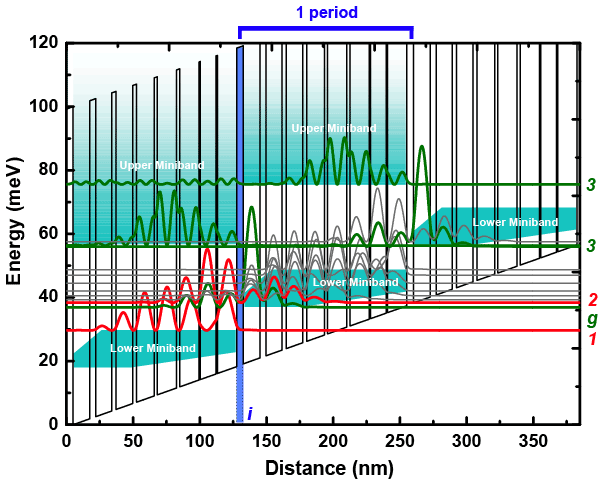Semiconductor Physics Group

# Longer wavelength QCLs

Because quantum cascade lasers operate on an intersubband transition, the energy, and hence frequency, of the laser may be engineered over a wide range.

We are currently working on extending this frequency range to lower frequencies by changing the design of our lasers. Below is the band diagram of a design that works at 2THz.

The black lines represent wells (GaAs) and barriers (AlGaAs) of the superlattice. The drawing shows 3 periods of superlattice, there are 90 in total. The superlattice is 'sloped' because there is an electric field of 1.9kV/cm applied. The wiggly lines are the electron wavefunctions, obtained by solving Schroedinger's equation. The red lines, labelled 1 & 2, are the lower and upper laser states, respectively. The green lines represent the ground-state wavefunctions for each miniband.